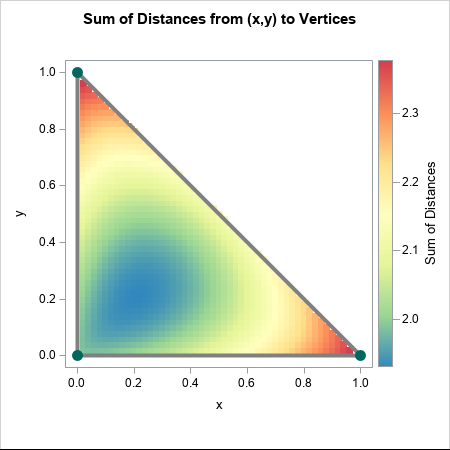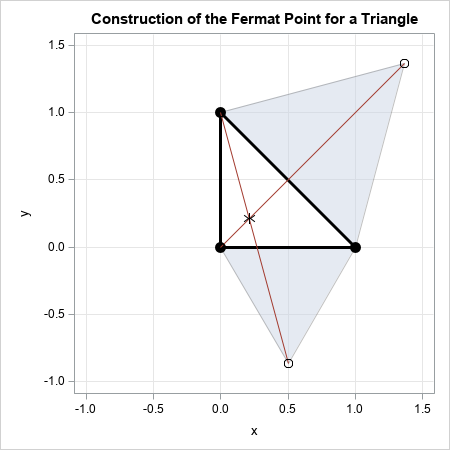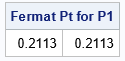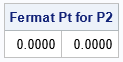While writing an article about labeling a polygon by using the centroid, I almost made a false claim about the centroid. I almost claimed that that the centroid is the point in a polygon that minimizes the sum of the distances to the vertices. It is not. The point that minimizes the distance to a set of points is called the geometric median of the set. There is no general formula or exact method for computing the geometric median of N points. In general, you need to use numerical optimization or another iterative technique to compute the geometric median.

However, for certain special cases, you can construct the geometric median directly. One special case is the triangle in the plane. For a triangle, the point that minimizes the sum of distances to the vertices is called the Fermat point (or sometimes the Fermat-Torricelli point). There is a geometric construction that enables you to find the exact geometric median (Fermat point) of a triangle. This article shows how to visualize the geometric median of a triangle and uses a SAS program to implement a geometric straightedge-and-compass construction that gives the exact location of the geometric median.### Visualize the Fermat point of a triangle

The easiest way to visualize the Fermat point is to compute the sum of the distances from every point, q, in a triangle to the three vertices. This is illustrated in the graph to the right, which shows the triangle with vertices (0,0), (1, 0), and (0,1). Each point in the interior of the triangle is colored according to the sum of the distances from the point to the vertices. For example, the sum of distances for the origin is 0 + 1 + 1 = 2, so the origin is assigned a green-blue color. For the other two vertices, the sum of the distances is 1 + 0 + sqrt(2) = 2.41, which is assigned a reddish color. You can see from the graph that points near (0.2, 0.2) appear to minimize the sum of distances for this triangle. The computation in this article shows that the exact location of the minimum is (0.2113, 0.2113).

### An algorithm for the Fermat point of a triangle

According to the Wikipedia article about the Fermat point of a triangle, you can use the following algorithm to compute the Fermat point. There are two cases:1. If the largest angle of the triangle exceeds 120 degrees, the Fermat point is the vertex that has the largest angle.
2. Otherwise, the Fermat point is in the interior of the triangle. Use the following geometric construction to compute the location:
• Choose two sides of the triangle. On each side, construct the equilateral triangle that lies entirely outside of the original triangle. Each equilateral triangle defines a new point that is not on the triangle.
• Draw a line from each new point to the opposite vertex of the original triangle.
• The two lines intersect at the Fermat point.

This algorithm is illustrated by the diagram to the right for the second case. The vertices of the original triangle are solid black circles. On two sides, I constructed an equilateral triangle. Each equilateral triangle defines a new point, which is shown as an unfilled circle. Red lines connect those points to the opposite vertex of the original triangle. The intersection of the lines is the Fermat point, which is shown as an asterisk.

### Compute the Fermat point of a triangle

Since there is an exact algorithm to compute the Fermat point, it makes sense to implement it. I will use the SAS IML matrix language to implement the computation of the geometric median of a triangle. According to the previous algorithm, you need to function that perform the following auxiliary tasks:

1. Construct an equilateral triangle on directed line segment in the plane. This is easy because the vertex of the equilateral triangle lies on the perpendicular bisector of the segment at a distance sqrt(3)/2 times the length of the segment. In the following program, this is implemented by the EquilateralPt function.
2. Find the intersection of two line segments. I have already written a function that computes the intersection of line segments. See the IntersectSegsSimple function.
3. Test whether the intersection point is inside the triangle. If so, you have found the Fermat point. You can use barycentric coordinates to test whether a point is inside a triangle. See the PtInTriangle function.
4. If the intersection point is not inside the triangle, return the vertex that has the largest angle. This is simply the angle opposite the largest side. See the VertexWithLargestAngle function.
```/* Construct the geometric median (Fermat point) of a triangle, which is "a point such that the sum of the three distances from each of the three vertices of the triangle to the point is the smallest possible." https://en.wikipedia.org/wiki/Fermat_point */ proc iml; /* Find 3rd vertex for equilateral triangle with vertices [x, y]. The vertex is on the right side of the directed segment from x to y. The vertex lies on the perpendicular bisector of the segment at a distance sqrt(3)/2 times the length of the segment. */ start EquilateralPt(x, y); m = (x + y)/2; /* middle of segment is the base of the perpendicular */ z = y - x; /* vector from x to y */ perp = z || -z; /* perpendicular vector of same length */ return( m + sqrt(3)/2 * perp ); finish;   /* Find the intersection of two line segments. S1 has endpoints p1 and p2. S2 has endpoints q1 and q2. See https://blogs.sas.com/content/iml/2018/07/09/intersection-line-segments.html */ start IntersectSegsSimple(p1, p2, q1, q2); b = colvec(q1 - p1); A = colvec(p2-p1) || colvec(q1-q2); /* nonsingular when segments have different slopes */ x = solve(A, b); /* x = (s,t) */ if all(0<=x && x<=1) then /* if x is in [0,1] x [0,1] */ return (1-x)*p1 + x*p2; /* return intersection */ else /* otherwise, segments do not intersect */ return ({. .}); /* return missing values */ finish;   /* compute barycentric coordinates for x in triangle whose vertices are the three rows of P. See https://blogs.sas.com/content/iml/2023/06/21/barycentric-coordinates.html */ start Barycentric(x, P); A = P` // j(1, nrow(P), 1); /* add constraint coefficients for sum(barycentric) */ b = x` // j(1,nrow(x),1); /* add constraint: sum(barycentric) = 1 */ v = solve(A, b); return( v` ); /* return a row vetor */ finish; /* For each input point, return 1/0 if the point is inside/outside the triangle whose vertices are the rows of P. */ start PtInTriangle(x, P); c = Barycentric(x, P); /* barycentric coordinates */ return( c[, ><] >=0 ); /* are all coords nonnegative? */ finish;   /* For triangle ABC, return the coordinates of the vertex that has the largest angle. This is the vertex that is opposite the longest side. */ start VertexWithLargestAngle(P); D = distance(P); /* matrix of distances of triangle edges */ dA = D[2,3]; /* length of side opposite from angle in triangle ABC */ dB = D[1,3]; dC = D[1,2]; /* you can use law of cosines if you want the actual angle */ if dA >= dB && dA >= dC then return( P[1,] ); if dB >= dA && dB >= dC then return( P[2,] ); return( P[3,] ); finish;   /* Construct the Fermat point in triangle P by using the algorithm at https://en.wikipedia.org/wiki/Fermat_point */ start FermatPt(P); p1 = EquilateralPt(P[1,], P[2,]); /* construct equilateral triangle on first side; p1 is new point */ p2 = P[3,]; /* opposite vertex */ q1 = EquilateralPt(P[2,], P[3,]); /* construct equilateral triangle on second side; p2 is new point */ q2 = P[1,]; /* opposite vertex */ *print p1, p2, q1, q2; v = IntersectSegsSimple(p1, p2, q1, q2); /* Fermat point is intersection of line segments */ if PtInTriangle(v,P) then /* is the Fermat point inside the triangle? */ return v; return VertexWithLargestAngle(P); /* otherwise, return vertex with largest angle */ finish; store module=(EquilateralPt IntersectSegsSimple Barycentric PtInTriangle VertexWithLargestAngle FermatPt); QUIT;```

With these functions defined, let's compute the Fermat point for the right isosceles triangle in the previous diagram:

```proc iml; load module=_all_;   /* test the algorithm on a right triangle */ P1 = {0 0, 1 0, 0 1}; v = FermatPt(P1); print v[F=6.4 L="Fermat Pt for P1"];```As indicated by the earlier graphs, the geometric median for this triangle is at (0.2113, 0.2113). This is the point in the triangle such that the sum of the distances to the vertices is a minimum.

Now let's compute the Fermat point for an obtuse triangle whose largest angle exceeds 120 degrees:

```/* test the algorithm on a triangle whose largest angle is > 120 deg */ P2 = {-2 1, 0 0, 1 0}; v = FermatPt(P2); print v[F=6.4 L="Fermat Pt for P2"];```For this triangle, the intersection point for the auxiliary line segments is outside of the triangle. Therefore, the Fermat point is the vertex of the largest angle, which is the vertex at (0,0).

### Summary

The geometric median of N points is the location for which the sum of the distances to the points is a minimum. In general, there is no explicit formula for the geometric median. However, if N=3 and the points are not collinear, then the geometric median is the classical Fermat point, which can be constructed by using a straightedge and a compass. This article uses SAS software to construct the geometric median (Fermat point) for a planar triangle.

Share